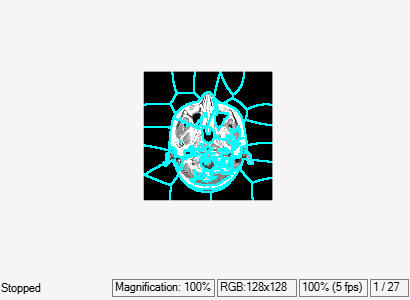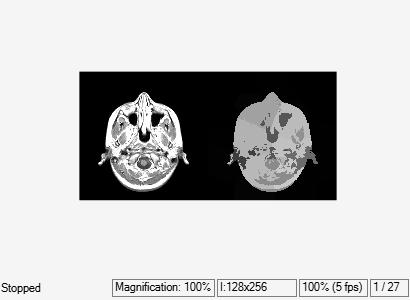# superpixels3

3-D superpixel oversegmentation of 3-D image

## Syntax

``````[L,NumLabels] = superpixels3(A,N)``````
``````[L,NumLabels] = superpixels3(___,Name,Value)``````

## Description

example

``````[L,NumLabels] = superpixels3(A,N)``` computes 3-D superpixels of the 3-D image `A`. `N` specifies the number of superpixels you want to create. The function returns `L`, a 3-D label matrix, and `NumLabels`, the actual number of superpixels returned.```
``````[L,NumLabels] = superpixels3(___,Name,Value)``` computes superpixels of image `A` using name-value pairs to control aspects of the segmentation.```

## Examples

collapse all

Load 3-D MRI data, remove any singleton dimensions, and convert the data into a grayscale intensity image.

```load mri; D = squeeze(D); A = ind2gray(D,map); ```

Calculate the 3-D superpixels. Form an output image where each pixel is set to the mean color of its corresponding superpixel region.

```[L,N] = superpixels3(A,34); ```

Show all xy-planes progressively with superpixel boundaries.

```imSize = size(A); ```

Create a stack of RGB images to display the boundaries in color.

```imPlusBoundaries = zeros(imSize(1),imSize(2),3,imSize(3),'uint8'); for plane = 1:imSize(3) BW = boundarymask(L(:, :, plane)); % Create an RGB representation of this plane with boundary shown % in cyan. imPlusBoundaries(:, :, :, plane) = imoverlay(A(:, :, plane), BW, 'cyan'); end implay(imPlusBoundaries,5) ```Set the color of each pixel in output image to the mean intensity of the superpixel region. Show the mean image next to the original. If you run this code, you can use `implay` to view each slice of the MRI data.

```pixelIdxList = label2idx(L); meanA = zeros(size(A),'like',D); for superpixel = 1:N memberPixelIdx = pixelIdxList{superpixel}; meanA(memberPixelIdx) = mean(A(memberPixelIdx)); end implay([A meanA],5); ```## Input Arguments

collapse all

Volume to segment, specified as a 3-D numeric array.

Data Types: `single` | `double` | `int8` | `int16` | `int32` | `uint8` | `uint16` | `uint32`

Desired number of superpixels, specified as a positive integer.

Data Types: `single` | `double` | `int8` | `int16` | `int32` | `int64` | `uint8` | `uint16` | `uint32` | `uint64`

### Name-Value Arguments

Specify optional pairs of arguments as `Name1=Value1,...,NameN=ValueN`, where `Name` is the argument name and `Value` is the corresponding value. Name-value arguments must appear after other arguments, but the order of the pairs does not matter.

Before R2021a, use commas to separate each name and value, and enclose `Name` in quotes.

Example: ```B = superpixels3(A,100,'NumIterations', 20);```

Shape of superpixels, specified as a numeric scalar. The compactness parameter of the SLIC algorithm controls the shape of the superpixels. A higher value makes the superpixels more regularly shaped, that is, a square. A lower value makes the superpixels adhere to boundaries better, making them irregularly shaped. You can specify any value in the range `[0 Inf)` but typical values are in the range `[0.01,0.1]`.

Note

If you specify the `'slic0'` method, you typically do not need to adjust the `'Compactness'` parameter. With the `'slic0'` method, `superpixel3` adaptively refines the `'Compactness'` parameter automatically, thus eliminating the need to determine a good value.

Data Types: `single` | `double` | `int8` | `int16` | `int32` | `int64` | `uint8` | `uint16` | `uint32` | `uint64`

Algorithm used to compute the superpixels, specified as one of the following values. For more information, see Algorithms.

Value

Meaning

`'slic0'`

`superpixels3` uses the SLIC0 algorithm to refine `'Compactness'` adaptively after the first iteration. This is the default.

`'slic'`

`'Compactness'` is constant during clustering.

Data Types: `char` | `string`

Number of iterations used in the clustering phase of the algorithm, specified as a positive integer. For most problems, it is not necessary to adjust this parameter.

Data Types: `single` | `double` | `int8` | `int16` | `int32` | `int64` | `uint8` | `uint16` | `uint32` | `uint64`

## Output Arguments

collapse all

Label matrix, returned as a 3-D array of positive integers. The value `1` indicates the first region, `2` the second region, and so on for each superpixel region in the image.

Data Types: `double`

Number of superpixels computed, returned as a positive number.

Data Types: `double`

## Algorithms

The algorithm used in `superpixels3` is a modified version of the Simple Linear Iterative Clustering (SLIC) algorithm used by `superpixels`. At a high level, it creates cluster centers and then iteratively alternates between assigning pixels to the closest cluster center and updating the locations of the cluster centers. `superpixels3` uses a distance metric to determine the closest cluster center for each pixel. This distance metric combines intensity distance and spatial distance.

The function's `Compactness` argument comes from the mathematical form of the distance metric. The compactness parameter of the algorithm is a scalar value that controls the shape of the superpixels. The distance between two pixels i and j, where m is the compactness value, is:

`$\begin{array}{l}{d}_{\mathrm{int}ensity}=\sqrt{{\left({l}_{i}-{l}_{j}\right)}^{2}}\\ {d}_{spatial}=\sqrt{{\left({x}_{i}-{x}_{j}\right)}^{2}+{\left({y}_{i}-{y}_{j}\right)}^{2}+{\left({z}_{i}-{z}_{j}\right)}^{2}}\\ D=\sqrt{{\left(\frac{{d}_{\mathrm{int}ensity}}{m}\right)}^{2}+{\left(\frac{{d}_{spatial}}{S}\right)}^{2}}\end{array}$`

Compactness has the same meaning as in the 2-D `superpixels` function: It determines the relative importance of the intensity distance and the spatial distance in the overall distance metric. A lower value makes the superpixels adhere to boundaries better, making them irregularly shaped. A higher value makes the superpixels more regularly shaped. The allowable range for compactness is `(0 Inf)`, as in the 2-D function. The typical range has been found through experimentation to be `[0.01 0.1]`. The dynamic range of input images is normalized within the algorithm to be from 0 to 1. This enables a consistent meaning of compactness values across images.

## Version History

Introduced in R2016b